## Example Questions

### Example Question #1 : Integers

When 8 integers are multiplied their product is negative, then at most how many of the integers can be negative?

5

4

7

1

8

7

Explanation:

When one multiplies two negative numbers (or any even multiple) the result is a positive number. However, when one multiplies three negative numbers (or any odd multiple) the product is negative. If the result of multiplying 8 negatives is odd, the largest number of negative integers will be the largest odd number, in this case 7.

### Example Question #5 : Even / Odd Numbers

If n and m are both positive even integers, which of the following must be odd?

I. (n + 1)(m + 1)

II. nm + 1

III. nm + m

I, II, and III

I and II only

II only

I only

II and III only

I and II only

Explanation:

Let us analyze I, II, and III one at a time.

Because n and m are both even, if we increase either by 1, the result will be an odd number. Thus, n + 1 and m + 1 are both odd. When two odd numbers are multiplied together, the result is always an odd number. Thus (n + 1)(m + 1) must be an odd number.

Because n and m are even, when we multiply two even numbers together, we always get an even number. Thus nm is even. However, when we then add one to an even number, the result will be an odd number. Thus, nm + 1 is odd.

We just established that nm is even. If we subtract an even number from an even number, the result is always even. Thus, nm – m is an even number.

Only choice I and II will always produce odd numbers.

The answer is I and II only.

### Example Question #41 : Integers

odd * odd * odd =

even * even * even

odd * odd

even * even

odd * odd * even

even * odd

odd * odd

Explanation:

The even/odd number properties are good to know. If you forget them, however, it's easy to check with an example.

Odd * odd = odd. If you didn't remember that, a check such as 1 * 3 = 3 will give you the same answer. So if odd * odd = odd, (odd * odd) * odd = odd * odd = odd, just as 3 * 3 * 3 = 27, which is odd. This means we are looking for an answer choice that also produces an odd number. Let's go through them.

even * even = even (2 * 2 = 4)

even * odd = even (2 * 3 = 6)

odd * odd = odd (1 * 3 = 3) This is the correct answer! But just to double check, let's go through the last two.

even * even * even = even * even = even (2 * 2 * 2 = 8)

odd * odd * even = odd * even = even (1 * 3 * 2 = 6)

### Example Question #42 : Integers

When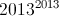is evaluated, what number is in the ones digit?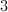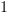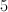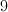Explanation:

Let us say we have two numbers,and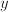whose ones digits are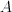and B, respectively. If we want to know the ones digits of the product ofand, all we need to do is to look at the ones digit of the product ofand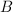. For example, if we multiply 137 and 219, then the ones digit will be the same as the ones digit of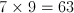. Since the ones digit of 63 is 3, the ones digit of 137 x 219 will also be 3. In short, we really only need to worry about the ones digits of the numbers we multiply when we try to find the ones digit of their product.

We want to find the ones digit of. An exponent is essentially just a short hand for repeated multiplication. Let us look at the ones digit of the first few exponents of 2013.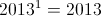- the ones digit is 3.

To find the ones digit of the 2013 to the second power, we need to think of it as the product of 2013 and 2013. As discussed previously, if we want the ones digit of two numbers multiplied together, we just need to multiply their ones digits. Thus, if we multiply 2013 by 2013, then the ones digit will be the same as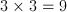.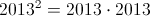- ones digit is 9.

Next, we want to find the ones digit of 2013 to the third power. In order to do this, we will multiply the square of 2013 by 2013. It does not matter that we do not know exactly what 2013 squared equals, beacuse we only need to worry about the ones digit, which is 9. In other words, 2013 to the third power will have a ones digit that is equal to the ones digit of the product of 9 (which was the ones digit of 2013 squared) and 3 (which is the ones digit of 2013). When we multiply 9 and 3, we get 27, so the ones digit of 2013 to the third power is 7.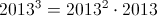- ones digit of 7.

To find the ones digit of 2013 to the fourth power, we only need to worry about multiplying the ones digit of 2013 to the third power (which is 7) by the ones digit of 2013. When we mulitply 7 and 3, we get 21, which means that the ones digit of 2013 to the fourth power is 1.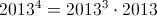- ones digit of 1.

To find the ones digit of 2013 to the fifth power, we will multiply 1 by 3, which gives us 3.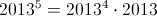- ones digit of 3.

Notice that we are back to a ones digit with 3. If we multiply this by 2013, we will end up with a ones digit of 9. In other words, the ones digits repeat every fourth power.

The value of the ones digits of the powers of 2013 is as follows (starting with 2013 to the first power):

3, 9, 7, 1, 3, 9, 7, 1,....

We essentially want to find the 2013th term of the sequence above. Notice that every fourth term is 1, i.e. the sequence repeats every four terms. If a terms position in the sequence is a multiple of 4, then the term will be 1. In short, the 4th, 8th, 12th, 16th terms, and so on, will be 1. Because 2012 is a multiple of 4, the 2012th term in the sequence will be 1. (We can determine if a number is a multiple of 4 by looking at its last two digits.) This means that that 2013th term will be 3. Thus, 2013 to the power of 2013 has a ones digit of 3.

### Example Question #1 : How To Multiply Odd Numbers

Ifandare both odd integers, which of the following is not necessarily odd?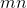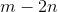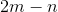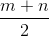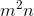Explanation:

With many questions like this, it might be easier to plug in numbers rather than dealing with theoretical variables.  However, given that this question asks for the expression that is not always even or odd but only not necessarily odd, the theoretical route might be our only choice.

Therefore, our best approach is to simply analyze each answer choice.:  Sinceis odd,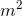is also odd, since and odd number multiplied by an odd number yields an odd product.  Sinceis also odd, multiplying it bywill again yield an odd product, so this expression is always odd.:  Sinceis odd, multiplying it by 2 will yield an even number.  Subtracting this number fromwill also give an odd result, since an odd number minus an even number gives an odd number.  Therefore, this answer is also always odd.:  Since both numbers are odd, their product will also always be odd.:  Sinceis odd, multiplying it by 2 will give an even number.  Sinceis odd, subtracting it from our even number will give an odd number, since an even number minus and odd number is always odd.  Therefore, this answer will always be odd.:   Since both numbers are odd, there sum will be even.  However, dividing an even number by another even number (2 in our case) does not always produce an even or an odd number.  For example, 5 and 7 are both odd.  Their sum, 12, is even.  Dividing by 2 gives 6, an even number.  However, 5 and 9 are also both odd.  Their sum, 14, is even, but dividing by 2 gives 7, an odd number.  Therefore, this expression isn't necessarily always odd or always even, and is therefore our answer.

### Example Question #2 : How To Multiply Odd Numbers

Theodore hasjelly beans. Portia has three times that amount. Harvey has five times as many as she does. What is the total count of jelly beans in the whole group?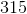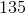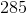Explanation:

To find the answer to this question, calculate the total jelly beans for each person:

Portia:* <Theodore's count of jelly beans>, which is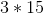orHarvey:* <Portia's count of jelly beans>, which is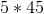or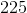So, the total is: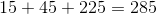(Do not forget that you need those originalfor Theodore!)

### Example Question #1 : How To Multiply Odd Numbers

If you multiply an even number by and odd number, what type of number will the answer be?

Zero

Even

A non-zero integer

Odd

Even

Explanation:

If you multiply an even number by and odd number, what type of number will the answer be?

For this question, let's try out a pair of numbers

Even: 6

Odd: 3

Now, if we multiply them, we get: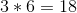18, an even number.

Any number multiplied by an even number will yield an even number.

### Example Question #1 : How To Multiply Odd Numbers

If two odd numbers are multiplied, what will the product be?

Odd

Even

Zero

Not enough information to solve

Odd

Explanation:

If two odd numbers are multiplied, what will the product be?

Let's begin by making up two numbers and seeing what we get.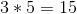So, we get 15, an odd number. Regardless of the two odd numbers we choose, we will always get an odd product.

### Example Question #1 : How To Multiply Odd Numbers

If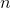is an odd integer, all of the following must be odd integers EXCEPT: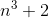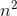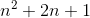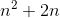Explanation:

Let's examine the choice. We can rewriteas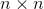, which would be multiplying an odd number (becauseis odd) by an odd number. Multiplying two odd numbers always produces another odd number. So this can't be the correct answer.

Next, let's look at. We can rewrite this as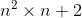. We already established thatmust be odd, so then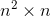must also be odd. If we then add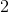to an odd number, we still get an odd number. So we can eliminate this choice as well.

Now, let's look at the choice. Let's factor this as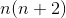. We know that n must be odd, and we know that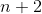must be odd. Therefore,is odd, because multiplying two odd numbers gives us an odd number.

Finally, let's analyze. We can rewrite this as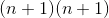. Since n is odd,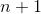must be an even number. When we multiply an even number by an even number, we get an even number, somust be even, and it cannot be odd.

The answer is.

### All SAT Math Resources